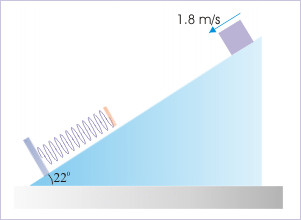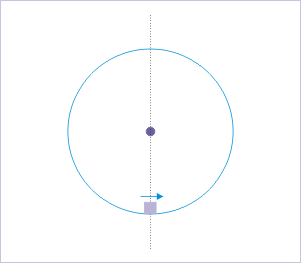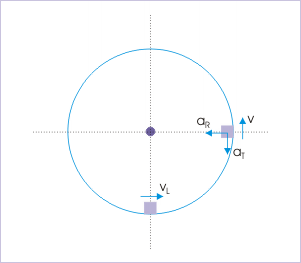# 13.14 Conservation of energy (application)  (Page 2/2)

 Page 2 / 2

$\Delta U={m}_{1}gh+{m}_{2}gh=4X10X2-10X10X2=-120\phantom{\rule{1em}{0ex}}J$

Now, the change in kinetic energy is given by :

$\Delta K=\frac{1}{2}{m}_{1}{v}^{2}+\frac{1}{2}{m}_{2}{v}^{2}$

$⇒\Delta K=\frac{1}{2}X4X{v}^{2}+\frac{1}{2}X10X{v}^{2}$

$⇒\Delta K=7{v}^{2}$

Now applying conservation of mechanical energy, we have :

$\Delta K+\Delta U=0$

$⇒7{v}^{2}-120=0$

$⇒v=4.14\phantom{\rule{1em}{0ex}}m/s$

Problem 4: The block of mass 10 kg is released in the arrangement consisting of various elements as shown in the figure. If spring constant is 50 N/m, then find the speed of 10 kg block, when block of 5 kg is about to leave the ground. (Consider g = 10 $m/{s}^{2}$ )

Solution : Before the block of 10 kg is released, the kinetic energy of the system is zero. We are required to find the speed of the released block, when the block in contact with horizontal surface is about to be lifted. It means that there is no change in kinetic or potential energy for the block of 5 kg.

In order to assess the energy state of the block of 10 kg, let us analyze the process. As the block of 10 kg moves down, it stretches the spring. As such, it acquires the speed (kinetic energy), while spring acquires elastic potential energy. However, at the same time, the gravitational potential energy of the block decreases as it comes down. Let the extension in the spring be “x” and let the speed of the mass of 10 kg be “v”, when block of 5 kg is about to be lifted.

Considering the system isolated, the change in kinetic energy is :

$\Delta K=\frac{1}{2}m{v}^{2}=\frac{1}{2}X10X{v}^{2}=5{v}^{2}$

The change in potential energy is :

$\Delta U=\frac{1}{2}k{x}^{2}-mgx$

We need to know “x” to evaluate the expression of potential energy. When the block of 5kg is about to be lifted, then force across the spring is equal to the difference of weights. Applying Hook’e law,

$Kx=\left(10-5\right)g=5X10=50$

$⇒x=\frac{50}{50}=1\phantom{\rule{1em}{0ex}}m$

Now, applying conservation of energy,

$\Delta U+\Delta K=0$

$⇒\frac{1}{2}k{x}^{2}-mgx+5{v}^{2}=0$

$⇒v=\frac{10X10X1-\frac{1}{2}X50X{1}^{2}}{5}$

$⇒v=15\phantom{\rule{1em}{0ex}}m/s$

## Incline – block - spring system

Problem 5: We are designing a delivery ramp for crates containing exercise equipment. The 1470-N crates will move at 1.8 m/s at the top of a ramp that slopes downward at 22 degrees. The ramp exerts a 550-N kinetic friction force on each crate, and the maximum static friction also has this value. Each crate will compress a spring at the bottom of the ramp and will come to rest after traveling a total distance of 8.0 meters along the ramp. Once stopped, a crate must not rebound back up the ramp. Calculate the maximum force constant of the spring which will meet the design criteria. Take sin22° = 0.374 and g = 10 $m/{s}^{2}$ .

This problem is adapted from the question mailed by a student.The block is moving with an initial speed down the incline.

Solution : Here the critical part of the question is to understand that the force required to return the crate up is equal to the sum of the component of its weight down the incline and the maximum static friction so that block can be initiated up the incline. Hence, spring force for this condition is :

${F}_{S}=mg\mathrm{sin}\theta +{F}_{K}=mg\mathrm{sin}{22}^{0}+550=1470X0.374+550=1100\phantom{\rule{1em}{0ex}}N$

Let “x” be the compression in the spring. Then, applying Hooke’ law, we get the compression in the spring as :

${F}_{S}=Kx=1100$

The system here is an isolated system with both conservative and non-conservative force. Hence,

${W}_{F}=\Delta K+\Delta U$

Here,

$⇒{W}_{F}=-550x8=-4400\phantom{\rule{1em}{0ex}}J$

$⇒\Delta K={K}_{f}-{K}_{i}=0-\frac{1}{2}X147X1.82=-238.14\phantom{\rule{1em}{0ex}}J$

$⇒\Delta U=\frac{1}{2}k{x}^{2}-mgh=\frac{1}{2}k{x}^{2}-1470X8X\mathrm{sin}{22}^{0}$

$⇒\Delta U=\frac{1}{2}k{x}^{2}-1470X8X\mathrm{sin}{22}^{0}=\frac{1}{2}k{x}^{2}-4398$

Putting values in the equation, we have :

$⇒{W}_{F}=\Delta K+\Delta U$

$⇒-4400=-238+\frac{1}{2}k{x}^{2}-4398$

$⇒\frac{1}{2}k{x}^{2}=238$

$⇒k{x}^{2}=476$

Combining with Hooke’s equation,

$⇒K=\frac{476{K}^{2}}{{1100}^{2}}$

$⇒K=\frac{{1100}^{2}}{476}=2542\phantom{\rule{1em}{0ex}}N/m$

We can see that a spring having spring constant less than this value will also not return the crate. Hence, this spring constant represents the maximum value of the spring constant, which meets the required design criterion.

## Vertical circular motion

Problem 5: An object inside a spherical shell is projected horizontally at the lowest point such that it just completes the circular motion in a vertical plane. If the radius of the shell is 2 m, then find the acceleration of the object when its velocity is vertical.An object inside a spherical shell is projected horizontally at the lowest point.

Solution : The object attains velocity in vertical direction, when it reaches half of the total height. The acceleration of the object is resultant of tangential and centripetal accelerations at that point :The acceleration of the object is resultant of tangential and centripetal accelerations.

$a=\sqrt{{a}_{T}^{2}+{a}_{R}^{2}}$

Here,

${a}_{T}=g$

and

${a}_{r}=\frac{{v}^{2}}{r}$

We need to know the speed of the object when it has reached half the height. Now, the minimum speed at the lowest point to enable to complete the revolution is :

${v}_{L}=\sqrt{5gr}$

From conservation of energy at lowest and middle levels,

${K}_{L}+{U}_{L}={K}_{M}+{U}_{M}$

Considering zero reference potential at the lowest point,

${U}_{L}=0$

${U}_{M}=mgr$

Putting these values in energy equation, we have :

$⇒\frac{1}{2}m{v}^{2}=\frac{1}{2}m{\left(\sqrt{5gr}\right)}^{2}-mgr$

$⇒{v}^{2}={\left(\sqrt{5gr}\right)}^{2}-2gr=3gr$

and

$⇒{a}_{r}=\frac{{v}^{2}}{r}=\frac{3gr}{r}=3g$

Thus,

$⇒a=\sqrt{\left\{{g}^{2}+{\left(3g\right)}^{2}\right\}}=\sqrt{10}g=10\sqrt{10}\phantom{\rule{1em}{0ex}}m/{s}^{2}$

Note : It is interesting to note that acceleration is independent of the radius of the shell.

List the application of projectile
pls explain what is dimension of 1in length and -1 in time ,what's is there difference between them
what are scalars
show that 1w= 10^7ergs^-1
what's lamin's theorems and it's mathematics representative
if the wavelength is double,what is the frequency of the wave
What are the system of units
A stone propelled from a catapult with a speed of 50ms-1 attains a height of 100m. Calculate the time of flight, calculate the angle of projection, calculate the range attained
58asagravitasnal firce
Amar
water boil at 100 and why
what is upper limit of speed
what temperature is 0 k
Riya
0k is the lower limit of the themordynamic scale which is equalt to -273 In celcius scale
Mustapha
How MKS system is the subset of SI system?
which colour has the shortest wavelength in the white light spectrum
if x=a-b, a=5.8cm b=3.22 cm find percentage error in x
x=5.8-3.22 x=2.58

#### Get Jobilize Job Search Mobile App in your pocket Now!By OpenStaxBy Steve GibbsBy Jonathan LongBy Brooke DelaneyBy OpenStaxBy Mucho MizindukoBy Dakota BocanBy OpenStaxBy Lakeima RobertsBy Michael Sag